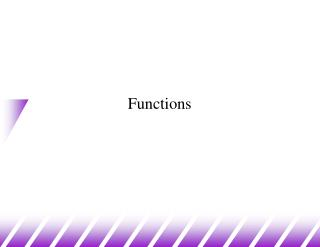# Functions - PowerPoint PPT PresentationDownload PresentationFunctions

Functions
Download Presentation## Functions

- - - - - - - - - - - - - - - - - - - - - - - - - - - E N D - - - - - - - - - - - - - - - - - - - - - - - - - - -
##### Presentation Transcript

1. Functions

2. int max(int a, int b); int main(){ int x; x = max(5,8); x = max(x,7); } int max(int a, int b){ return a>b?a:b; } Example

3. What is a C Function? • A function receives zero or more parameters, performs a specific task, and returns zero or one value. • A function is invoked by its name and parameters. • No two functions have the same name in your C program. • No two functions have the same name AND parameter types in your C++ program. • The communication between the function and invoker is through the parameters and the return value. • A function is independent: • It is “completely” self-contained. • It can be called at any places of your code and can be ported to another program. • Functions make programs reusable and readable.

4. Syntax • Function Prototype: return_type function_name (type1 name1, type2 name2, ..., typen namen); • Function Definition: return_type function_name (type1 name1, type2 name2, ...,typen namen) { ....statements... } The parameters Function header

5. void parameter list means it takes no parameters return type void means it returns nothing Some Examples • Function Prototype Examples double squared (double number); void print_report (int); int get _menu_choice (void); • Function Definition Examples double squared (double number) { return (number * number); } void print_report (int report_number) { if (report_nmber == 1) printf(“Printer Report 1”); else printf(“Not printing Report 1”); }

6. Passing Arguments • Arguments are passed as in Java and Pascal • Function call: func1 (a, b, c); • Function header int func1 (int x, int y, int z) • Each argument can be any valid C expression that has a value: • For example: x = func1(x+1,func1(2,3,4),5); • Parameters x y z are initialized by the value of a b c • Type conversions may occur if types do not match.

7. All parameters are passed by value!! This means they are basically local variables initialized to the values that the function is called with. They can be modified as you wish but these modifications will not be seen in the calling routine! #include<stdio.h> int twice(int x) { x=x+x; return x; } int main() { int x=10,y; y=twice(x); printf("%d,%d\n",x,y); } Parameters are Passed by Value

8. Returning a Value • To return a value from a C function you must explicitly return it with a return statement. • Syntax: return <expression>; • The expression can be any valid C expression that resolves to the type defined in the function header. • Type conversion may occur if type does not match. • Multiple return statements can be used within a single function (eg: inside an “if-then-else” statement…)

9. Local Variables • Local Variables int func1 (int y){ int a, b = 10; float rate; double cost = 12.55; .......} • Those variables declared “within” the function are considered “local variables”. • They can only be used inside the function they were declared in, and not elsewhere.

10. A Simple Example #include <stdio.h> int x=1; /* global variable - bad! */ void demo(void); int main() { int y=2; /* local variable to main */ printf ("\nBefore calling demo(), x = %d and y = %d.",x,y); demo(); printf ("\nAfter calling demo(), x = %d and y = %d.\n",x,y); return 0; } void demo () { int x = 88, y =99; /* local variables to demo */ printf ("\nWithin demo(), x = %d and y = %d.",x,y); }

11. For large programs Manage related functions in a .c file Write a .h file containing all the prototypes of the functions #include the header file in the files that uses the functions. For small programs, use the following order in the only one file: All prototypes main() function Other functions mymath.h int min(int x,int y); int max(int x,int y); mymath.c int min(int x,int y) { return x>y?y:x; } int max(int x,int y) { return x>y?x:y; } Placement of Functions

12. unsigned int factorial(unsigned int a); int main () { unsigned int f,x; printf("Enter value between 1 & 8: "); scanf("%d", &x); if (x > 8 || x < 1) printf (”Illegal input!\n"); else { f = factorial(x); printf ("%u factorial equals %u\n", x,f); } } unsigned int factorial (unsigned int a) { if (a==1) return 1; else { a *= factorial(a-1); return a; } } Recursion - An Example